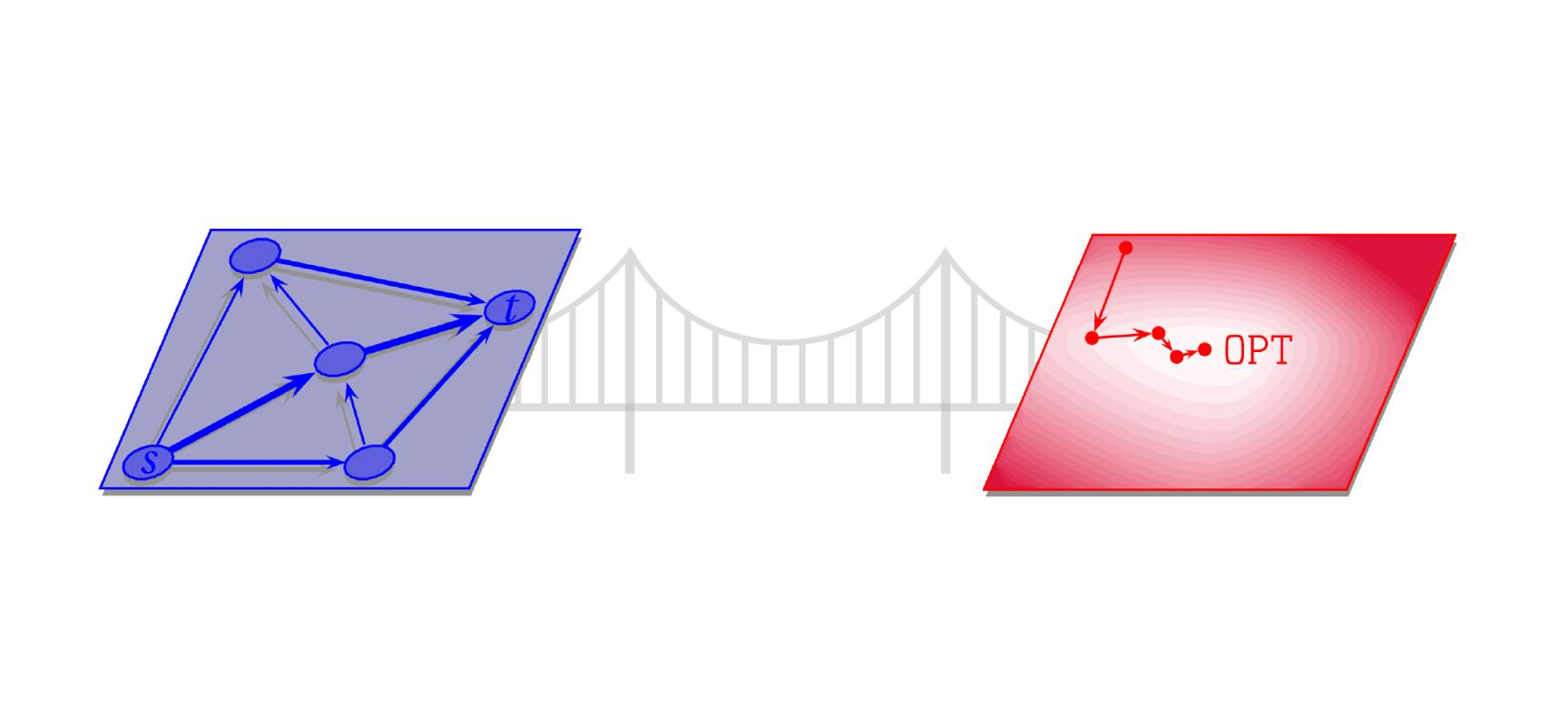# Looking Ahead: Bridging Continuous and Discrete OptimizationIn the coming fall semester, the Simons Institute will host a program on Bridging Continuous and Discrete Optimization. For the first time, instead of having two programs running in parallel during a semester (or in staggered terms, as with the Information Theory program and the Cryptography program in the spring and summer of 2015), we will have a single program with roughly double the usual number of visitors and postdoctoral fellows.

The program, organized by Aleksander Mądry, Jan Vondrák, Nikhil Bansal, Nick Harvey, Seffi Naor, Lorenzo Orecchia, Pablo Parrilo, Thomas Rothvoß and Ben Recht, will kick off with a series of lectures during the third week of August. During the program, there will be four thematic workshops, exploring the overarching theme of connections between discrete and continuous optimization.

Traditionally, discrete optimization has been a core part of the study of algorithms and complexity in theoretical computer science, while research on continuous optimization was carried out by electrical engineers and numerical analysts.

One point of contact between these two domains has been the study of continuous convex relaxations of discrete problems. In some cases, such as maximum flow and bipartite matching, the continuous relaxation delivers an integral optimal solution for the discrete problems. In most other cases, the optimum of the relaxation is different from the optimum of the discrete problem, but one can round the optimum of the relaxation to an approximate discrete solution. For many interesting relaxations, it remains an open problem to characterize the approximation ratio that can be achieved in such a way. For example, it is believed that the Held-Karp relaxation provides a constant-factor approximation for the Asymmetric Traveling Salesman Problem, but the best-known rounding scheme achieves an approximation ratio polynomial in loglogn, where n is the number of cities.

Two of the four workshops of the program will focus on the power and limitations of convex relaxations of discrete problems. One workshop, held in September, will be about rounding schemes and approximation algorithms. A later workshop, held in November, will be about impossibility results, showing that certain classes of linear programming and semidefinite programming relaxations cannot provide a good approximation for certain problems.

A more recent synthesis of discrete and continuous approaches to optimization originates from the work of Spielman and Teng on solving Laplacian systems of linear equations in nearly linear time. Spielman and Teng work within the classical (in the numerical analysis literature) approach of reducing the problem of solving a linear system with a positive semidefinite constraint matrix to an unconstrained minimization problem, and to devise a preconditioner to speed up the convergence of gradient descent methods applied to the resulting minimization problem. Their innovation is to introduce graph-theoretic techniques, such as low-stretch spanning trees and spectral sparsifiers, to devise such a preconditioner.

In turn, their nearly-linear time algorithm for solving Laplacian linear equations can be used to compute, in nearly-linear time, graph-theoretic quantities, such as the electrical flow in a network, or the effective resistance of the edges of a network. The ability to efficiently compute such quantities has played a key role in recent algorithmic advances for various graph problems, including max flow.

A workshop held in October will focus on iterative methods in continuous optimization, such as gradient descent and interior point methods, the application of techniques from the discrete algorithms domain to speed up iterative methods (as in the cases mentioned above), and the applications of iterative methods to discrete problems.

Another thread that runs across recent research on optimization is the realization that worst-case analysis and approximation-ratio analysis are not the best ways to understand the performance of algorithms in certain application domains. For example, in machine learning, one formulates the task of training a model or discovering features as an optimization problem, and the inputs to the optimization problem are i.i.d. samples from a distribution that is unknown but about which one can make reasonable assumptions. In such a case, an average-case analysis of the behavior of the algorithm might yield much better guarantees than a worst-case analysis. The last workshop of the program, held in December, will focus on optimization problems whose inputs are randomized, including the case in which part of the input is unknown, or is discovered by the algorithm in an online fashion.

,